# Working with Percentages

Data Pipeline

### How can I calculate a percentage?

Percentages can be used to gain valuable insight into the affects a portion has on a total. They are heavily relied upon to determine and evaluate KPIs for an organization and guide operations to improve your overall business performance.

In this example, we’re dividing our cancelled subscriptions over our total subscriptions to calculate our churn rate. Churn rate is a common metric used by SaaS companies to determine how effectively they are retaining their customers.

First we will need to create a dataset to calculate the number of customers who have not cancelled. To do this we will count the number of subscriptions where the payment start date is not null and the cancelled date is null.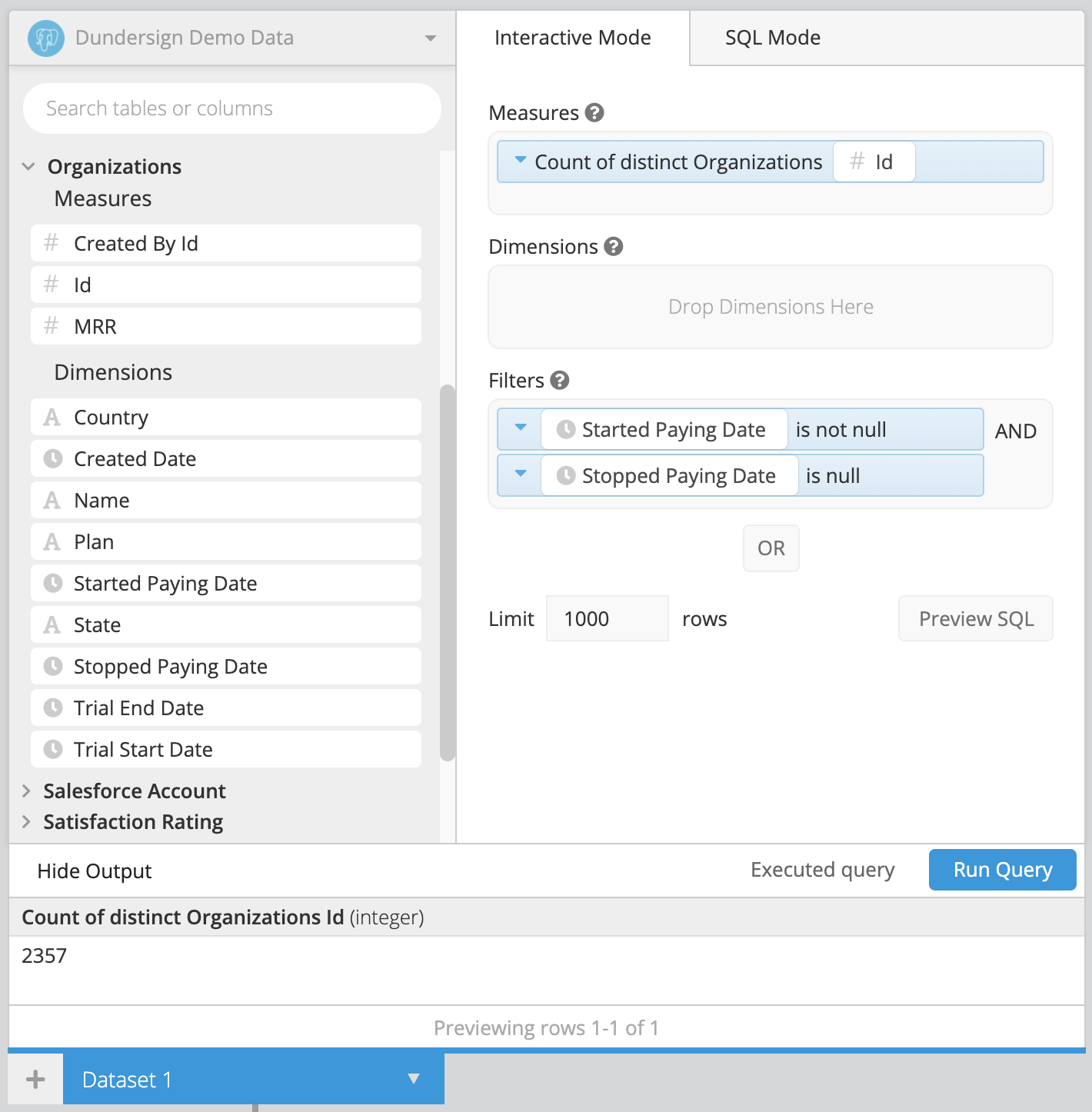Dataset 2 will get a count of subscriptions that have cancelled. To achieve this we will count the number of subscriptions where both the payment start date and the cancelled date is null.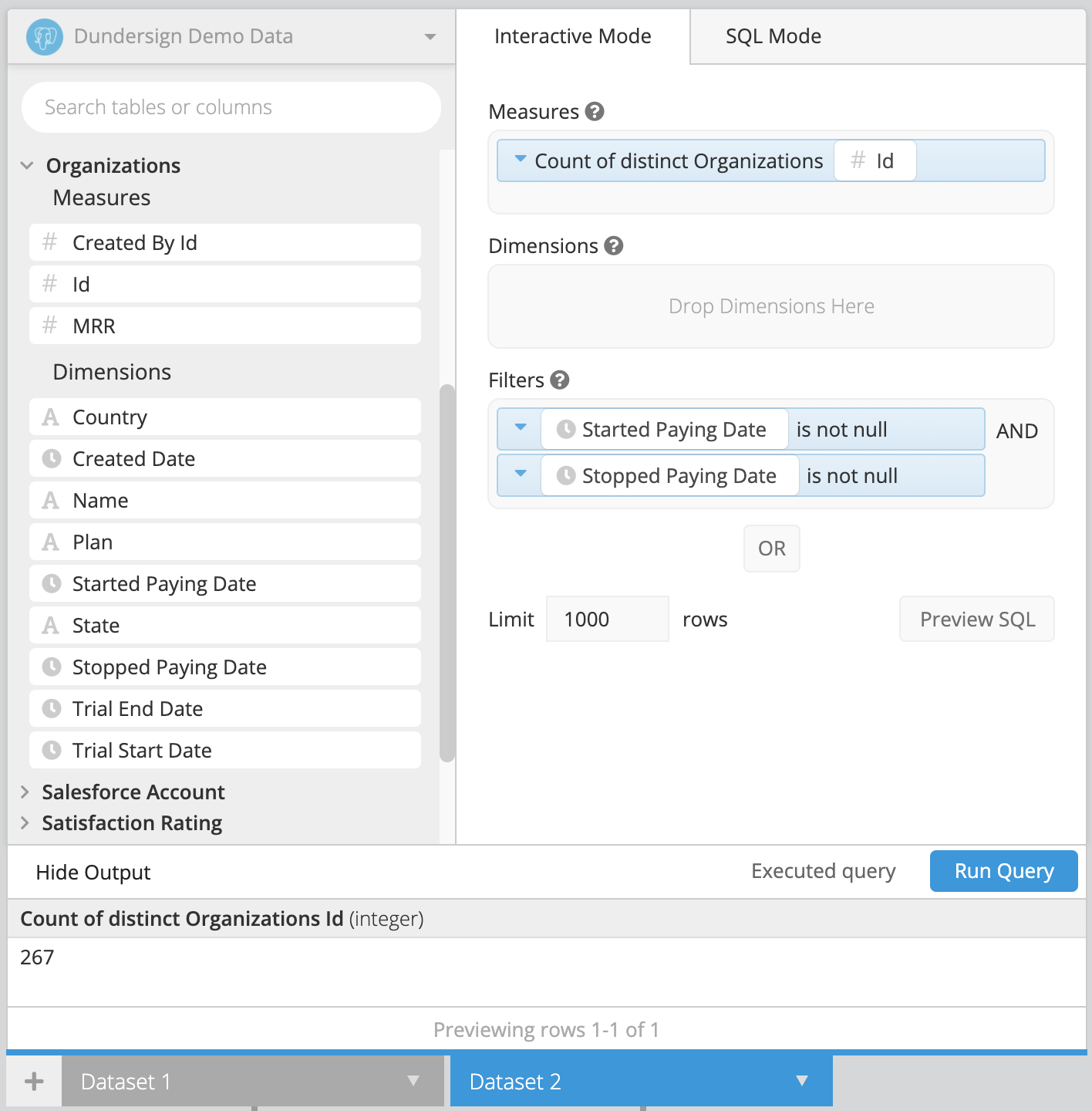After you’ve created your two datasets, make sure to use a Cross Join.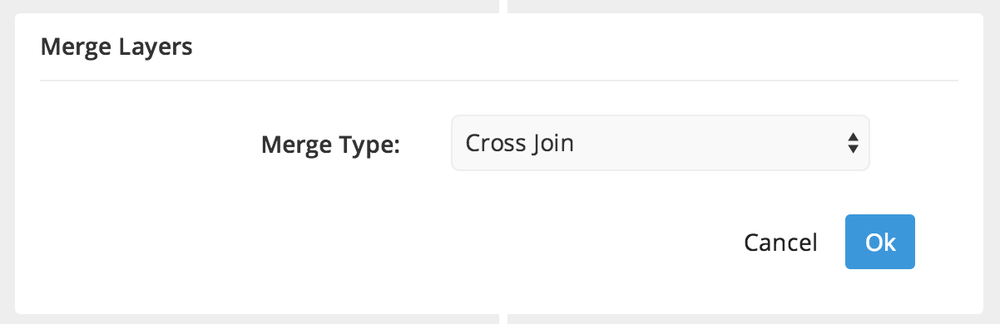In the Data Pipeline, click +Add Step, then choose Add Column from the dropdown menu. Set a title for your column, then enter the percentage formula, which is as follows:

``````100.0 * "Column1" / "Column2"
``````

To round your percentage to two decimal points, you can use the SQLite round() function. Your formula will look like this:

``````round( 100.0 * "Column1" / Column2", 2)
``````

Where 2 is the number of decimal places.

If your column values are integers and you would like your results to have decimal precision, be sure to multiply by 100.0 to convert the column over to a float data type. This will convert the top value to a decimal before the division step, and will ensure your results are in decimal form.

Our example looks as follows: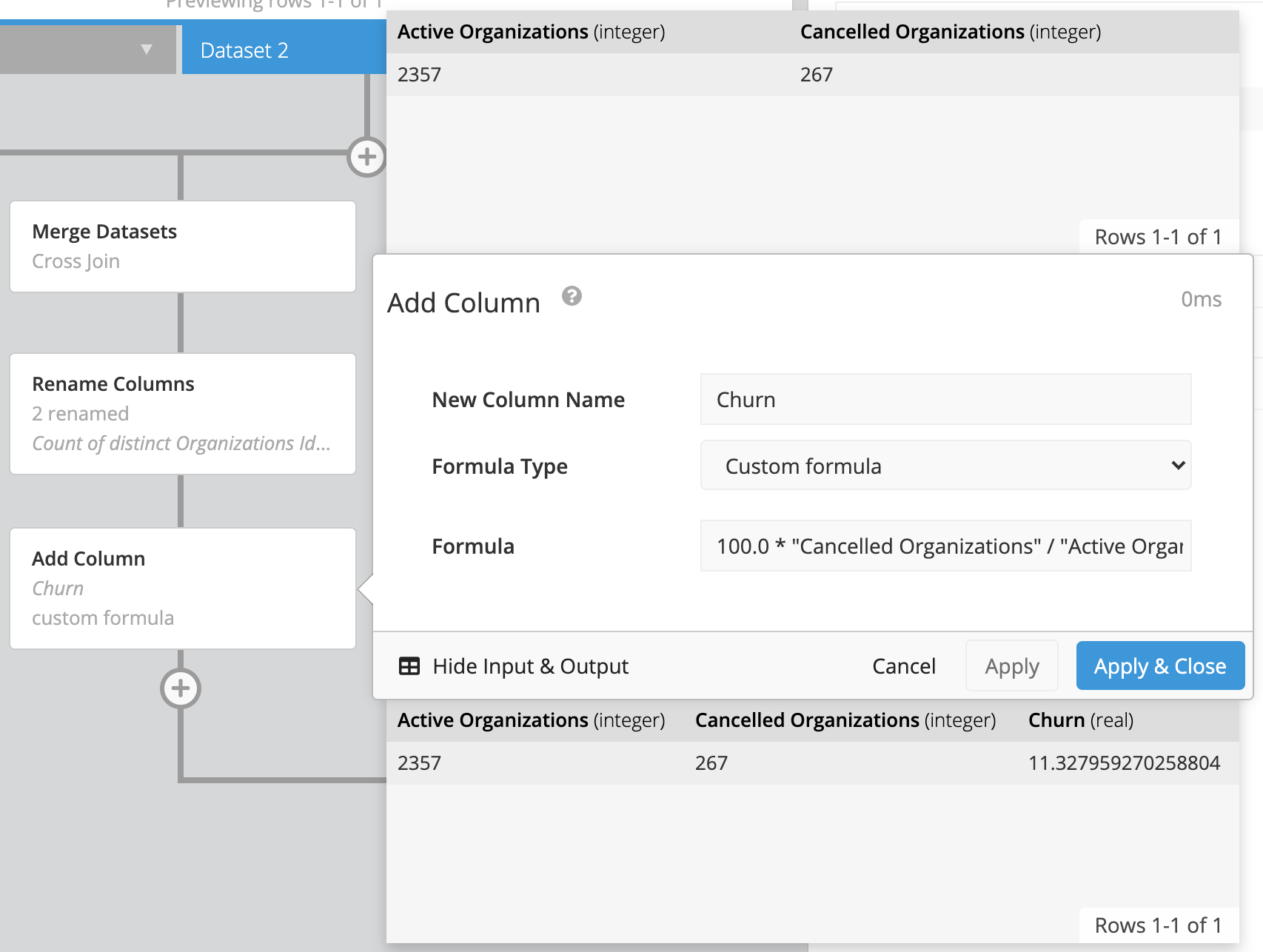Click Ok, and your percentages will be calculated.

### How to Troubleshoot Percent Change

If you’re using the Percent Change formula in one of your Data Pipeline steps, you may notice that if the previous row is 0, the percent change will return a NULL result. Don’t worry this is expected and this article will help you deal with those situations.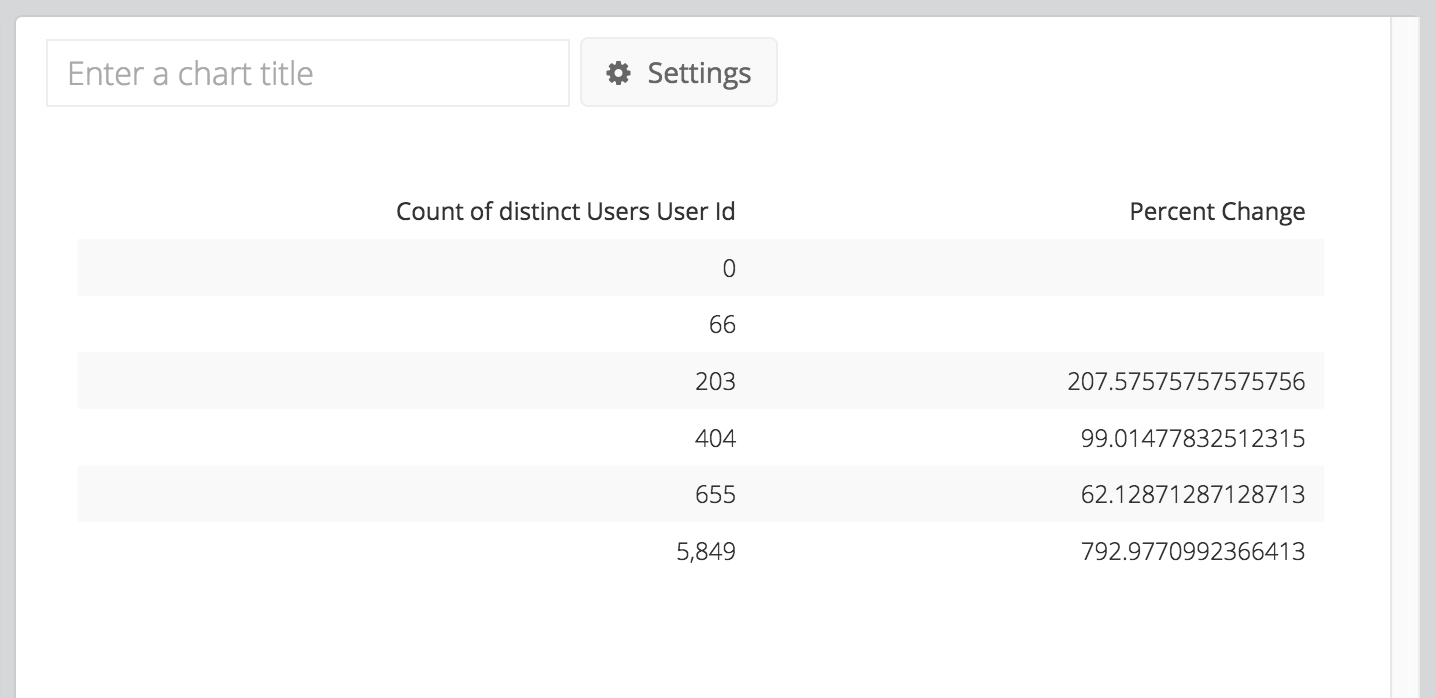Our Data Pipeline runs on SQLite which adheres to Postel’s Rule. The default behavior when dividing by zero is to return NULL rather than throw an error when dividing by zero (see SQLite documentation for more information). This lines up with the generally accepted principle that the concept of percentage change has no meaning essentially when starting from zero.

There are several articles online showing potential workarounds. In Chartio however, one would be to add an Edit Column step after the percent change step to denote that the percent change doesn’t apply here. You would select a custom formula in your Pipeline step and add the following CASE statement to it, replacing “Percent Change” with the name of your column:

``````case when "Percent Change" is null then 'N/A' else "Percent Change" end
``````

See more